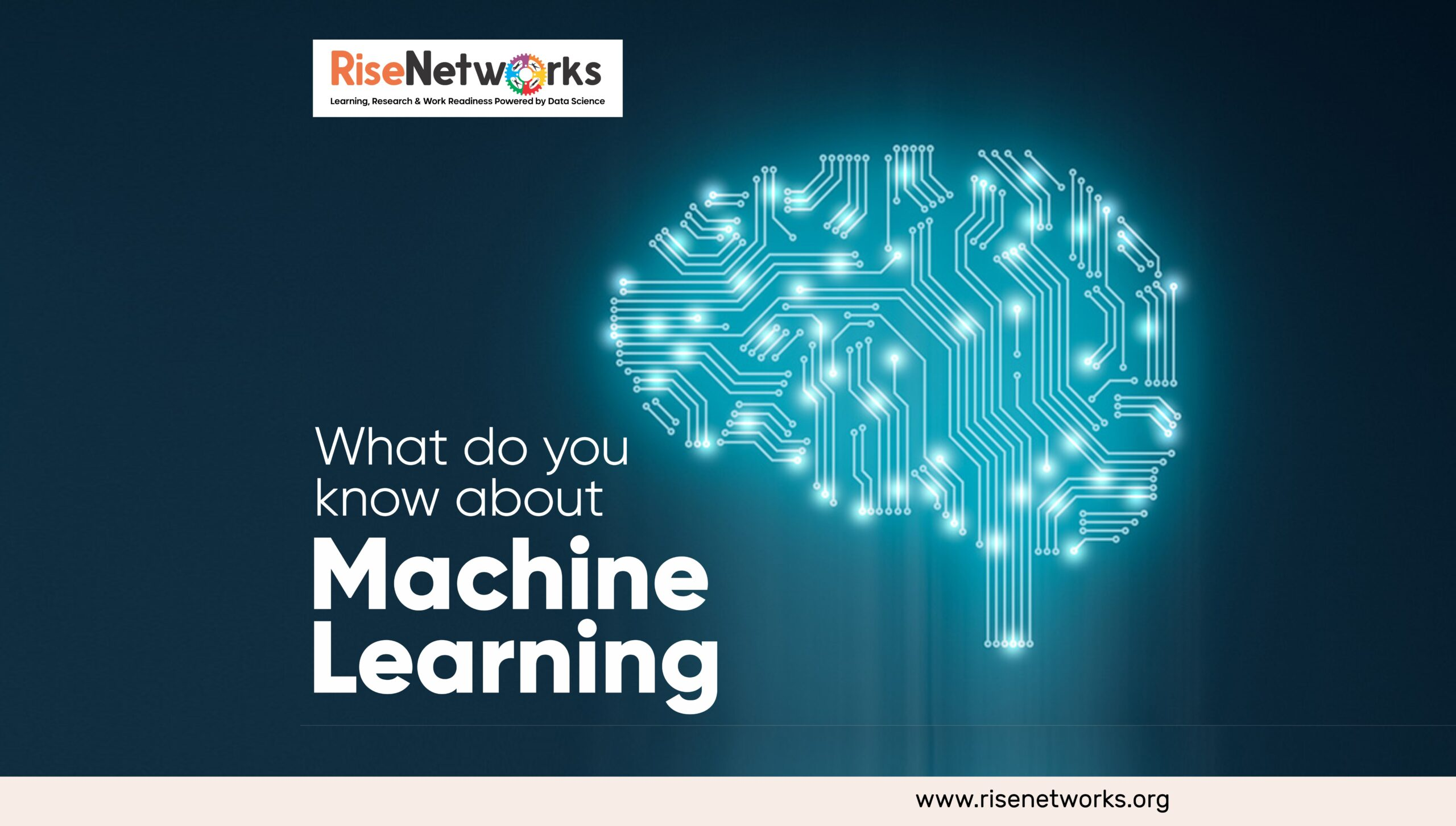Rise Networks# What do you know about Machine Learning?First time I read about Machine Learning, I thought it was a robot or something futuristic that might happen later in the future but you know what? Machine Learning is here. Machine learning has actually been around for a while, in fact Artur Samuel was the first person to use the term “Machine Learning” in 1959 where he defined it as a field of study that gives computers the ability to learn without being explicitly programmed.

What is Machine Learning?

Machine Learning is simply the process of programming a computer to make precise decisions. Machine Learning is divided into Supervised Learning and Unsupervised Learning.

• Supervised Learning occurs when the machine is trained with labeled data(data inputted into the machine) the machine learns from the labelled data and uses that knowledge to make decisions. For example if you want a machine to identify a car, you input different types and sizes of cars into the machine as samples, these samples are called labelled data. Then the machine learns from the sample and uses that to identify a car in future. Examples of Supervised Learning Algorithms are Linear regression, K-Nearest Neighbor

• Unsupervised Learning occurs when the machine deals with unlabeled data, the machine is expected to work on its own to discover information, this model doesn’t require supervision. Using the sample in supervised learning above, if we want our machine to identify a car, we input different random objects into the machine, the machine sorts the data on its own and classifies each appropriately.

Tools used for Machine Learning.

Different languages and frameworks are used for machine learning, they include Python, Javascript, Java,C++, R and TypeScript. Python is the most popular language used in Machine learning. Python Frameworks used for Machine Learning include Pandas, Numpy, Seaborn, Scipy and Scikit-learn, etc. I will explain what each is used for below.

• Pandas: This is a fast, powerful, flexible and easy to use data analysis and manipulation tool. To install Pandas, go to your terminal or command prompt and type “ pip install pandas” note that you need to have python installed before you can use pip.

• Numpy: Numpy is a numerical library in Python, It can be used to perform a number of mathematical operations on arrays such as trigonometric, statistical, and algebraic routine. Numpy forms the basis of powerful machine learning libraries like scikit-learn and SciPy. To install Numpy, go to your terminal or command prompt and type “ pip install numpy”

• Seaborn: Seaborn is a data visualization library in python,It is used to create for drawing attractive and informative statistical graphics. To install Seaborn, go to your terminal or command prompt and type “ pip install seaborn”

• Scipy: Scipy is built on Numpy for more mathematical operation, It uses numpy as the basic data structure dnd comes with modules for various commonly used tasks in scientific programming. To install Scipy, go to your terminal or command prompt and type “ pip install scipy”

• Scikit-Learn: This library is maximised by Machine Learning Engineers, it is used for algorithm transformation and prediction, It provides many unsupervised and supervised learning algorithms. Scikit-Learn can be installed from the terminal or command prompt using “pip install sklearn”
0